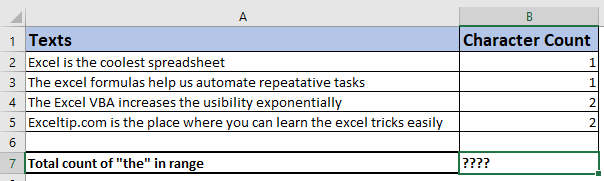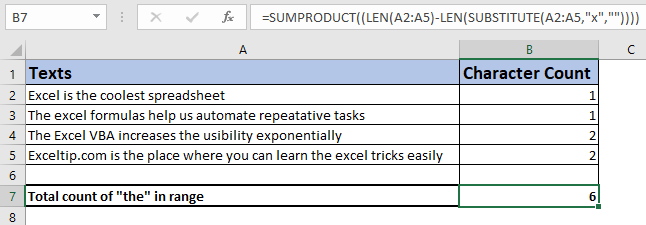# How to Count Specific Character in a Range

We know that the total characters in a cell can be counted using the Excel LEN function. We also have learned how to count total characters in a range. Now to count the total number of specific text in range (more than one cell), we will use a similar technique.

There are two methods to of doing this. Let's take a look at the first method.

Generic formula for counting characters in a range

 =SUMPRODUCT(LEN(range)-LEN(SUBSTITUTE(range,"character","")))

range: It is the range that contains the text.

character: This is the character that you want to count. It can be a specific character or a sentence.

Example: Count specific text in a rangeIn the image above, we have some texts in range B3:B6. We need to count the total number of "x" character in the range using one formula. We have manually written to the number of "x"s in each cell to check the result. We can sum them up but we want to use one formula that does not depend on another columns or manual work. We use the generic formula mentioned above.

Write this formula in cell C8.

 =SUMPRODUCT(LEN(B3:B6)-LEN(SUBSTITUTE(B3:B6,"x",""))))

Hit enter. We have a total count of characters in the range, which is 6.How does it work?

The SUMPRODUCT function is an array function that sums up the given array. The LEN function returns the length of the string in a cell or given text. SUBSTITUTE function returns an altered string after replacing a specific character with another.

Now the function runs inside out. So we first take the LEN(B3:B6) part.

Here we have fed the LEN function with a range of B3:B6. The LEN function returns the length of each cell in an array.

 {32;53;51;69}

Next LEN(SUBSTITUTE(B3:B6,"x","")). Here, the SUBSTITUTE function runs and returns an array of altered strings after replacing all "x"s with nothing ("") from the range B3:B6. Then that array is fed again to LEN function that returns the number remaining characters. {31;52;49;67}

We currently have two arrays in the SUMPRODUCT function, =SUMPRODUCT({32;53;51;69}-{31;52;49;67}). The second array is subtracted from first array and we get a final array as, =SUMPRODUCT({1;1;2;2}).  And finally this array is summed up to give final result as 6.

Case insensitive formula

The above formula is case sensitive, because the SUBSTITUTE function is a case sensitive function. To count specific character in range irrespective of it's case, use this formula.

 =SUMPRODUCT(LEN(B3:B6)-LEN(SUBSTITUTE(LOWER(B3:B6),"x",""))))

In this formula, LOWER function converts all string in range B3:B6 to lower case and then whole process happens, as discussed above.

Alternative Formula:

The alternative way is use of SUM function with LEN function as array formula. It means that we need to use CTRL+SHIFT+ENTER key combination after writing the function.

 {=SUM(LEN(B3:B6)-LEN(SUBSTITUTE(B3:B6,"x",""))))}

Counting specific text in a range:

You may be we wondering now how to count specific text in a range. That can be done with a minor change in the formula that is discussed here.

So yeah guys, this is how you can get total counts of specific character in a range.  This is quite easy but if you have any doubts or any other special query related to this article or any other Excel 2010/2013/2016/2019/365 or excel VBA, write it in the comments section below. I will be pleased to clear you doubts.

Related Articles:

Count Characters in a Cell in Excel | Learn how to count specific character in a cell or string.

Count total matches in two ranges in Excel | Learn how to count total matches in two ranges using SUMPROUDCT function.

SUMIFS using AND-OR logic | The SUMIFS can be used with OR logic too. The defualt logic SUMIFS uses is AND logic.

SUMPRODUCT with IF logic | Learn how to use SUMPRODUCT with IF logic without using IF function in the formula.

Popular Article:

50 Excel Shortcuts to Increase Your Productivity | Get faster at your task. These 50 shortcuts will make you work even faster on Excel.

The VLOOKUP Function in Excel | This is one of the most used and popular functions of excel that is used to lookup value from different ranges and sheets.

COUNTIF in Excel 2016 | Count values with conditions using this amazing function. You don't need filter your data to count specific value. Countif function is essential to prepare your dashboard.

How to Use SUMIF Function in Excel | This is another dashboard essential function. This helps you sum up values on specific conditions.

Terms and Conditions of use

The applications/code on this site are distributed as is and without warranties or liability. In no event shall the owner of the copyrights, or the authors of the applications/code be liable for any loss of profit, any problems or any damage resulting from the use or evaluation of the applications/code.